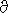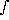# Potential Temperature

Derived directly from integration of the 1st law of thermodynamics. It is the temperature a parcel of air at P and T would have if it were at Ps. It is conserved for adiabatic motions, ( i.e., d/dt = 0).

dQ/(n · <mw> · T) = (Cp dT/T) - (Rg dP/P) = 0= T · (Ps/P)= P/(Rg) [(Ps/P)]= Rg/Cp

For earth= 0.286 (<mw> = 28.96, Cp = 1.004 Joules/gram/K). Some authors write this equation with= Cp/Cv = 1/(1-)P/z = -· g = (P · g)/(Rg · T) = - P/H(z)log(P) = -H(z) ·z
therefore,
P/Ps = e-dz'/H(z')= T · e-dz'/H(z')
if H(z) = H0= T · e-· z/H0